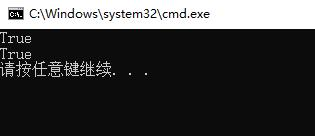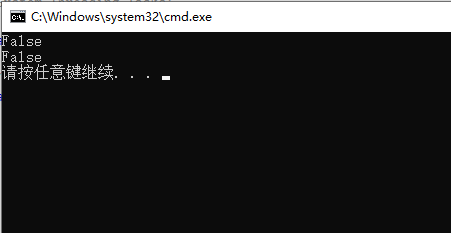# 1.值类型的比较

static void Main(string[] args)
{
int a = 100;
int b = 100;
Console.WriteLine(a == b);
Console.WriteLine(a.Equals(b));

}# 2.引用类型的比较

class Program
{
static void Main(string[] args)
{
MyInt myInt1 = new MyInt();
myInt1._a = 100;
myInt1._b = 'a';
MyInt myInt2 = new MyInt();
myInt2._a = 100;
myInt2._b = 'a';
Console.WriteLine(myInt1==myInt2);
Console.WriteLine(myInt1.Equals(myInt2));

}
}
public class MyInt
{
public int _a;
public char _b;
}# 3.String类型的==和Equals

static void Main(string[] args)
{
String str1 = "abc";
String str2 = "abc";
Console.WriteLine(str1 == str2);
Console.WriteLine(str1.Equals(str2));

}